Function Repository Resource:

# MalfattiCircles

Construct the Malfatti circles of a triangle

Contributed by: Minh Trinh Xuan
 ResourceFunction["MalfattiCircles"][{p1,p2,p3}] constructs the Malfatti circles of the triangle with corner points p1,p2, and p3.

## Details

The three Malfatti circles of a triangle are tangent to the sides of the triangle and each other.
ResourceFunction["MalfattiCircles"][Triangle[{p1,p2,p3}]] is equivalent to ResourceFunction["MalfattiCircles"][{p1,p2,p3}].

## Examples

### Basic Examples (2)

Find the Malfatti circles of a triangle:

 In:=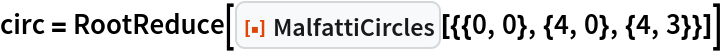Out=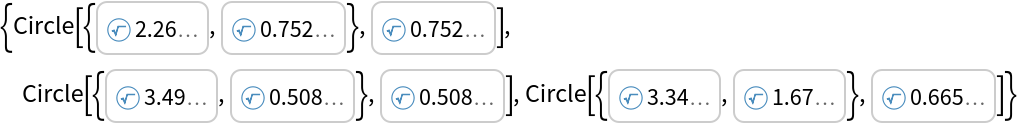Show the circles:

 In:=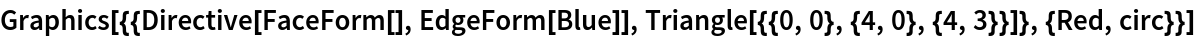Out=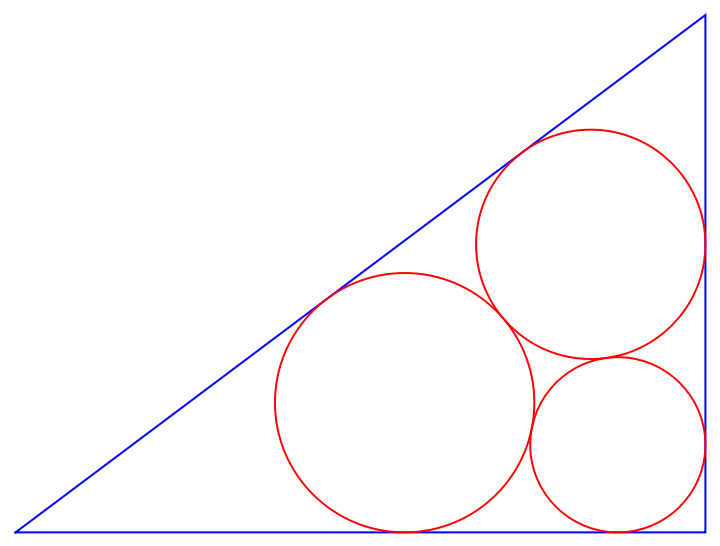### Scope (2)

Find the Malfatti circles of a Triangle object:

 In:=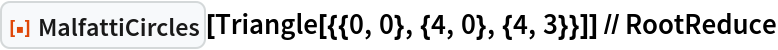Out=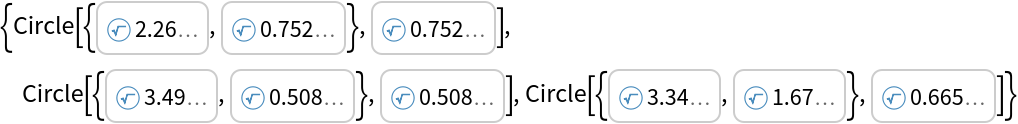Malfatti circles with integer coordinates and radii:

 In:=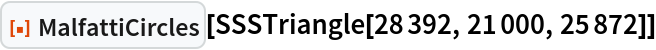Out=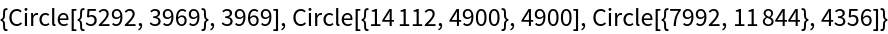### Properties and Relations (1)

The inradius of a triangle can be expressed in terms of the radii of the Malfatti circles:

 In:=Out=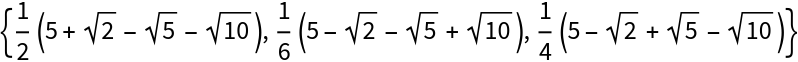In:=Out=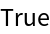### Neat Examples (2)

Show the Malfatti circles of an arbitrary triangle in a Manipulate:

 In:=Out=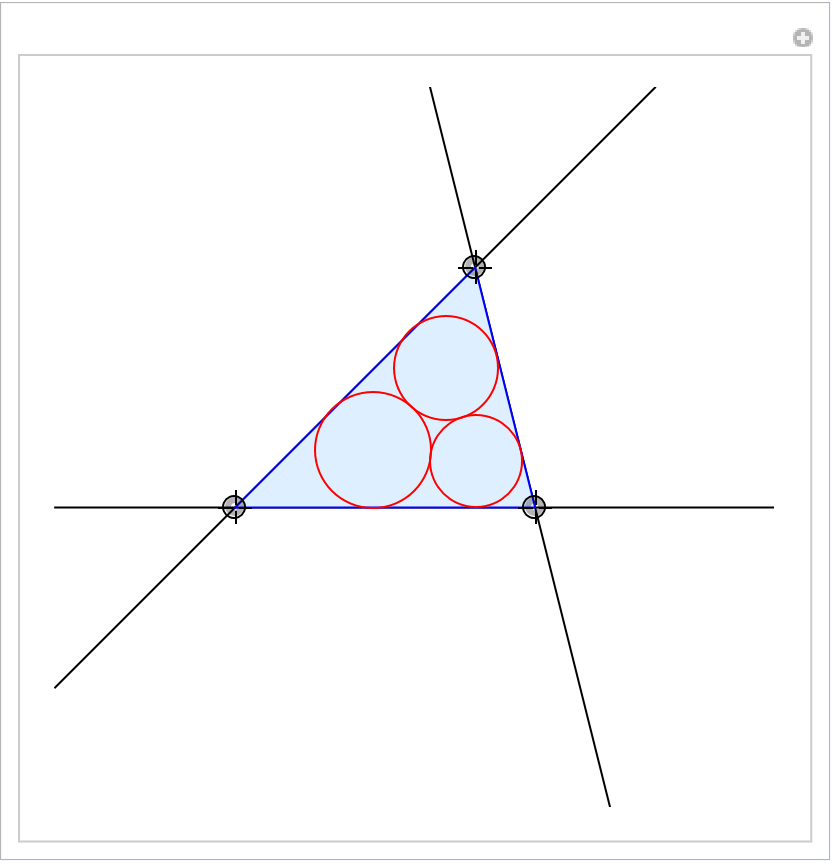Iteratively form the Malfatti circles of the triangle formed by the centers of the excircles:

 In:=Out=Trinh Xuan Minh

## Version History

• 1.0.0 – 22 August 2022